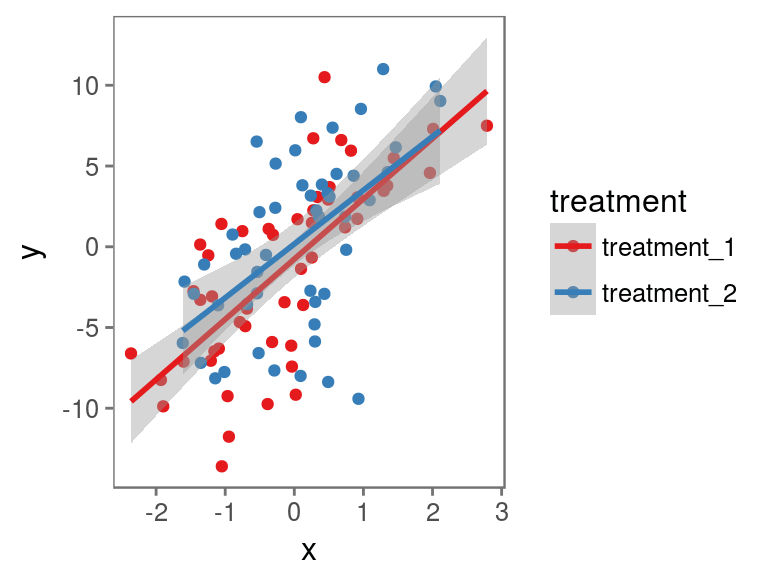9th January 2017

## Overview

• Why now?
• Why R?
• General tips
• Recommended packages
• Recommended resources

## Why now?

### Efficiency

• Point-and-click software just isn't time efficient
• Automating tasks will pay off within the time frame of a PhD and thereafter

## Why now?

### Reproducibility

• There is an increasing expectation that materials, data, and analysis details are provided alongside research to ensure it is reproducible
• This is easier when things are script based
• Peer Reviewers' Openness Initiative

## Why R?

### Jobs

• R is increasingly taught in Psychology departments, including at undergraduate level
• Useful skill for jobs outside academia
• Makes you a more efficient academic

## Why R?

### Pretty graphs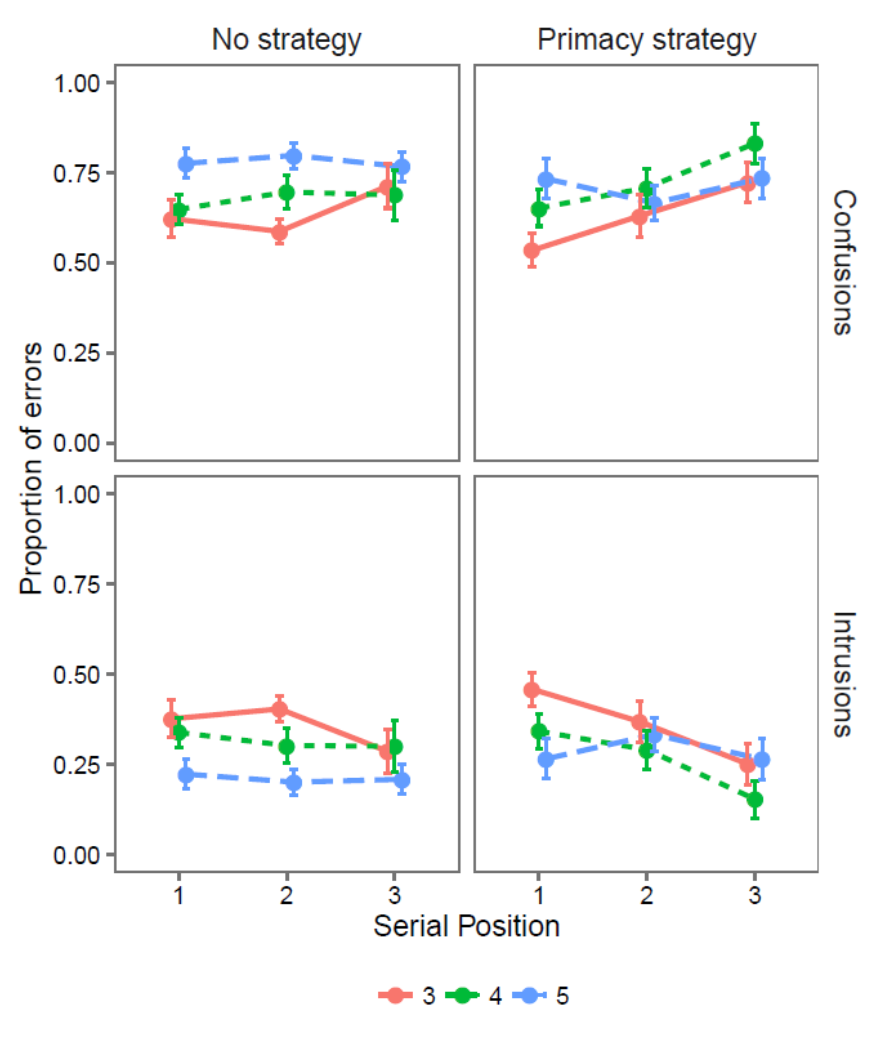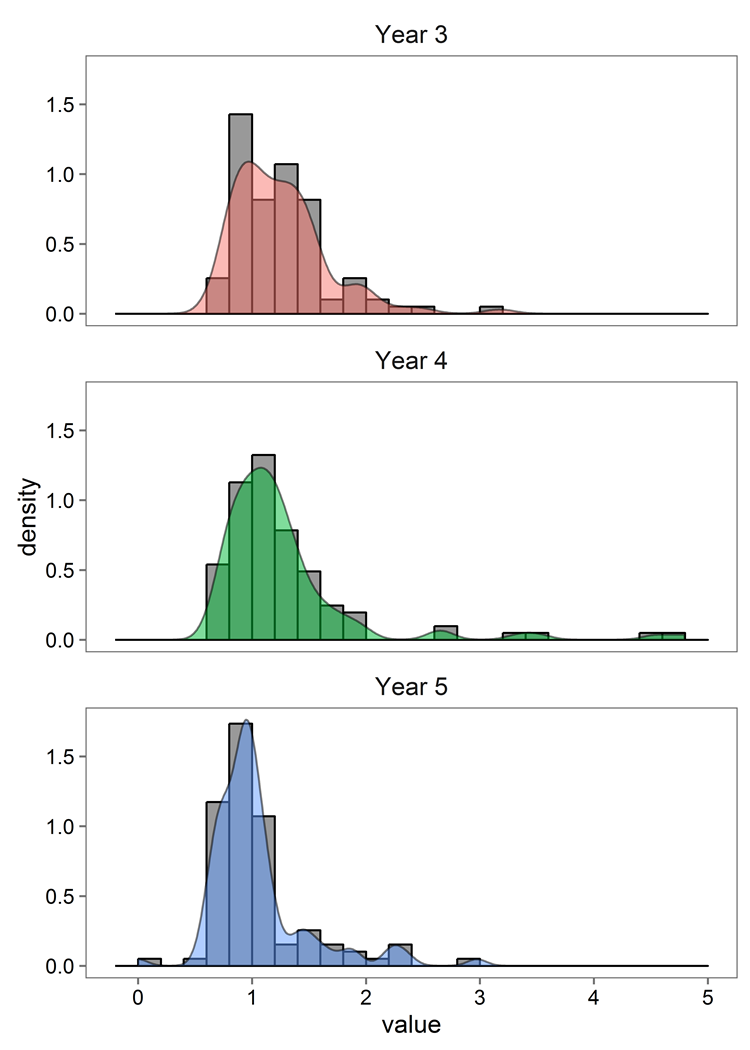## Why R?

### Range of packages

• There are R packages for a huge range of analyses
• Great data manipulation packages
• Slides
• Documents
• Including books
• Interactive HTML applications

• R projects
• R Markdown

## Recommended packages

• Given the age of R there are many ways to complete a task
• Most data manipulation tasks can be done with 'base R'
• However, this often isn't the most efficient or readable approach

## tidyverse

• A collection of packages by Hadley Wickham for:
• Data visualisation (ggplot2)
• Data manipulation (dplyr)
• Data tidying (tidyr)
• Functional programming (purrr)
• See here for a full list of the included packages
• These packages are all designed to work nicely together
• More readable by people than most R code

## Installing tidyverse

• To install and load any package you just do:
```install.packages("tidyverse")
library(tidyverse)```
• You need to load a package in with `library()` for any new R session you want to use it with

## ggplot2

• Build graphs by specifying:
• Aesthetics: physical properties of the plot mapped to variables in the data (x & y positions, size, shape, colour etc.)
• Geometries: what to actually use to represent the data (lines, bars, points etc.)

## ggplot2

### qplot

`qplot(x = df\$x, y = df\$y)`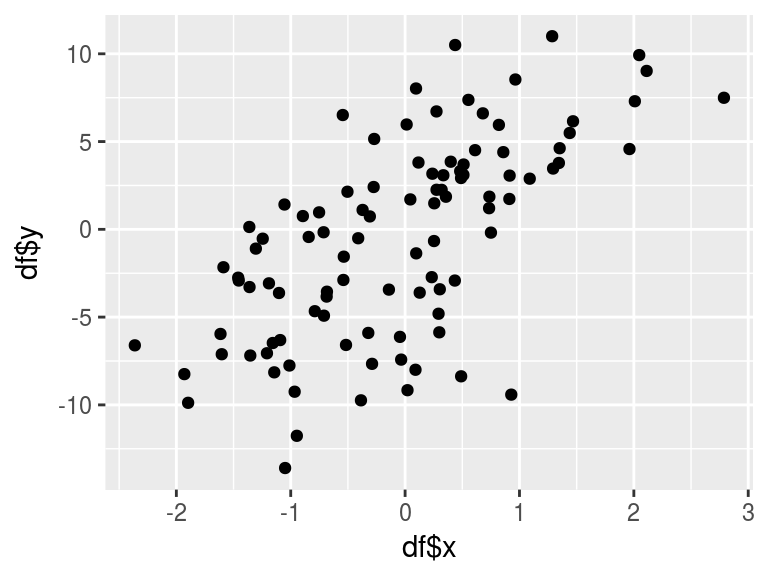## ggplot2

### qplot

```qplot(df\$x, df\$y) +
geom_smooth(method = "lm")```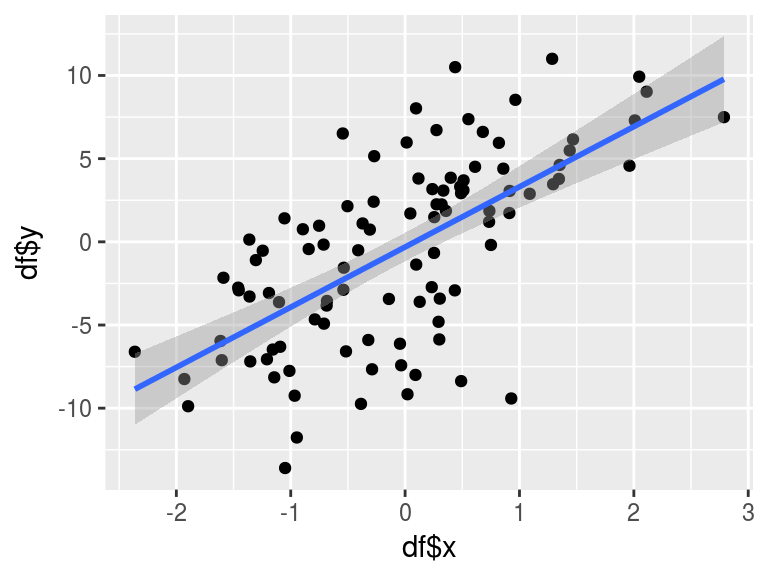## ggplot2

### ggplot

```ggplot(data = df, mapping = aes(x = x, y = y)) +
geom_point() +
geom_smooth(method = "lm")```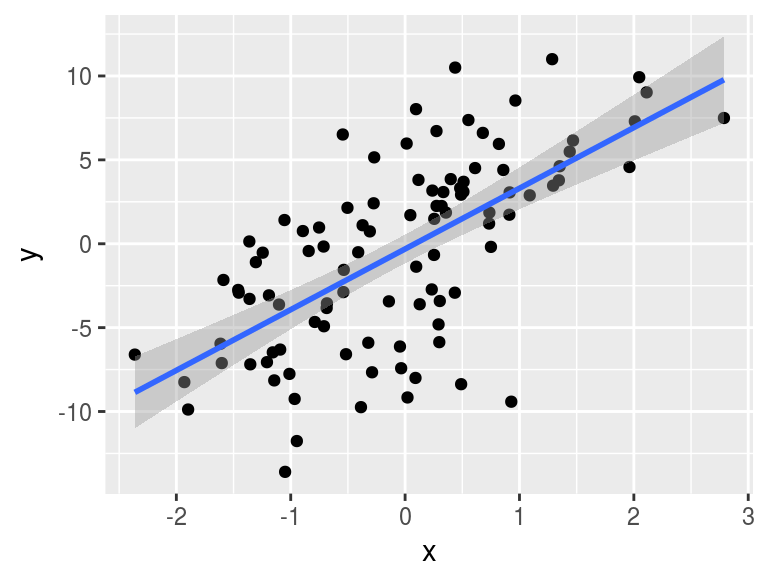## ggplot2

### ggplot

```ggplot(data = df, mapping = aes(x = x, y = y, colour = treatment)) +
geom_point() +
geom_smooth(method = "lm")```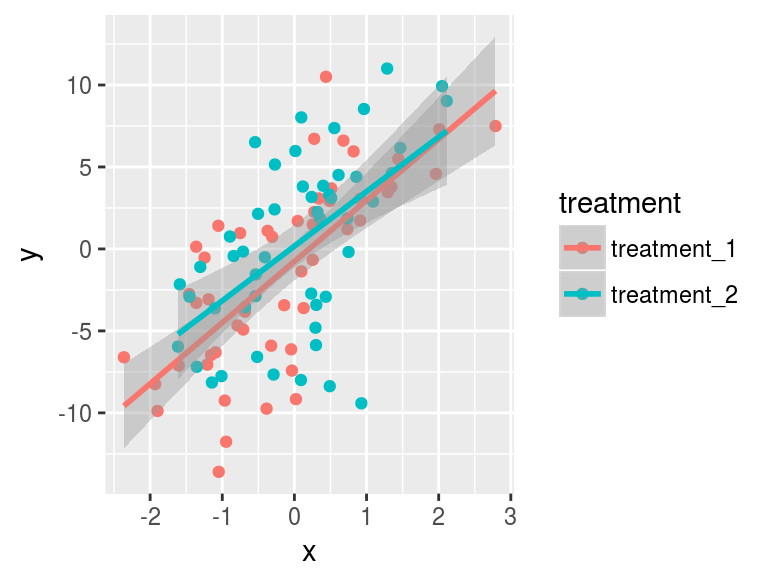## ggplot2

### Other tips

• The package `ggthemes` is good for providing premade plot 'styles'
• `RColorBrewer` is useful for colours
• Useful info on colour in `ggplot2` here
• `cowplot` is good for creating grids of labelled plots for papers

## ggplot2

### Other tips

```ggplot(df, aes(x, y, colour = treatment)) +
geom_point() +
geom_smooth(method = "lm") +
theme_few() +
scale_color_brewer(palette = "Set1")```